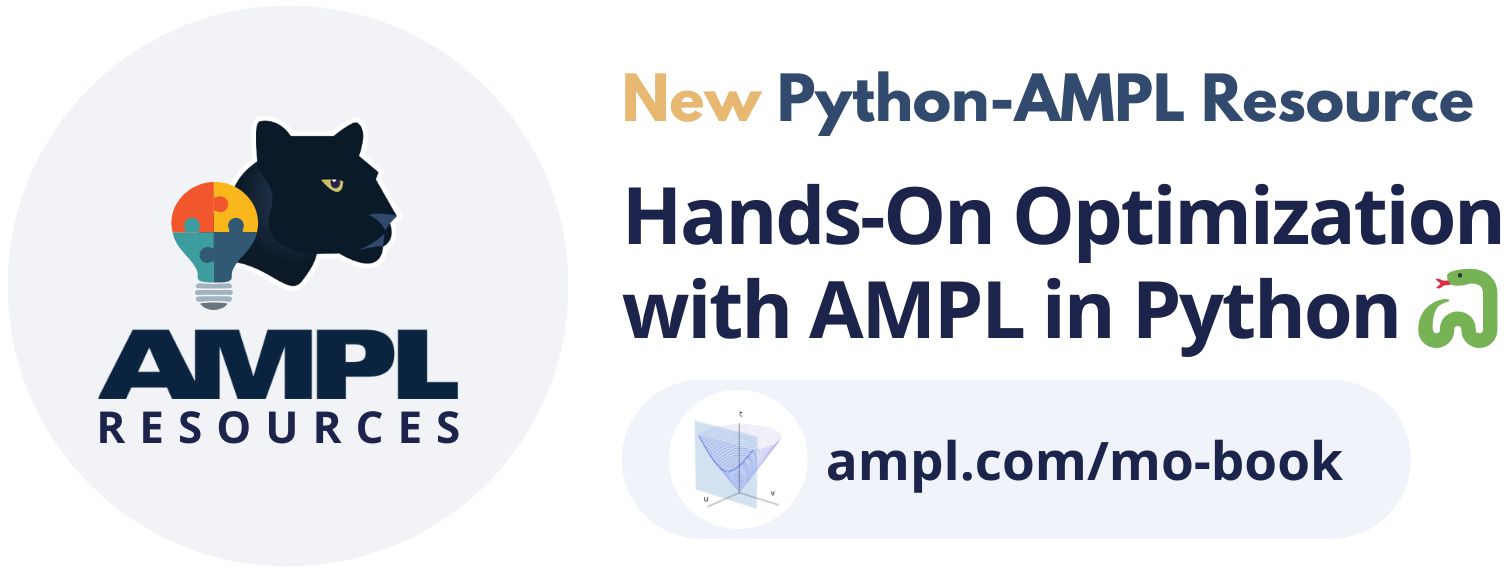# AMPL Python API¶

`amplpy` is an interface that allows developers to access the features of AMPL from within Python. For a quick introduction to AMPL see Quick Introduction to AMPL.

In the same way that AMPL’s syntax matches naturally the mathematical description of the model, the input and output data matches naturally Python lists, sets, dictionaries, `pandas` and `numpy` objects.

All model generation and solver interaction is handled directly by AMPL, which leads to great stability and speed; the library just acts as an intermediary, and the added overhead (in terms of memory and CPU usage) depends mostly on how much data is sent and read back from AMPL, the size of the expanded model as such is irrelevant.

With `amplpy` you can model and solve large scale optimization problems in Python with the performance of heavily optimized C code without losing model readability. The same model can be deployed on applications built on different languages by just switching the API used.Many more notebooks with examples are available on the AMPL Model Colaboratory and the new book Hands-On Optimization with AMPL in Python 🐍.

## Installation & minimal example¶

```# Install Python API for AMPL
\$ python -m pip install amplpy --upgrade

# Install solver modules (e.g., HiGHS, CBC, Gurobi)
\$ python -m amplpy.modules install highs cbc gurobi

\$ python -m amplpy.modules activate <license-uuid>

# Import in Python
\$ python
>>> from amplpy import AMPL
>>> ampl = AMPL() # instantiate AMPL object
```

Note

You can use a free Community Edition license, which allows free and perpetual use of AMPL with Open-Source solvers.

```# Minimal example:
from amplpy import AMPL
import pandas as pd
ampl = AMPL()
ampl.eval(r"""
set A ordered;
param S{A, A};
param lb default 0;
param ub default 1;
var w{A} >= lb <= ub;
minimize portfolio_variance:
sum {i in A, j in A} w[i] * S[i, j] * w[j];
s.t. portfolio_weights:
sum {i in A} w[i] = 1;
""")
tickers, cov_matrix = # ... pre-process data in Python
ampl.set["A"] = tickers
ampl.param["S"] = pd.DataFrame(
cov_matrix, index=tickers, columns=tickers
)
ampl.option["solver"] = "gurobi"
ampl.option["gurobi_options"] = "outlev=1"
ampl.solve()
assert ampl.solve_result == "solved"
sigma = ampl.get_value("sqrt(sum {i in A, j in A} w[i] * S[i, j] * w[j])")
print(f"Volatility: {sigma*100:.1f}%")
# ... post-process solution in Python
```